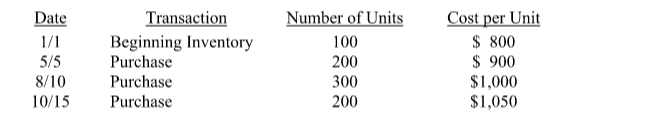# Problem: Lauer Corporation uses the periodic inventory system and the following information about their laptop computer is available: During the year, 750 laptop computers were sold. What was ending inventory and cost of goods sold on 12/31 under the LIFO cost flow assumption? a. $56,000 and$714,000. b. $45,000 and$725,000. c. $40,000 and$730,000. d. None of the answers is correct.

###### Problem Details

Lauer Corporation uses the periodic inventory system and the following information about their laptop computer is available:

During the year, 750 laptop computers were sold.

What was ending inventory and cost of goods sold on 12/31 under the LIFO cost flow assumption?

a. $56,000 and$714,000.

b. $45,000 and$725,000.

c. $40,000 and$730,000.

d. None of the answers is correct.Frequently Asked Questions

What scientific concept do you need to know in order to solve this problem?

Our tutors have indicated that to solve this problem you will need to apply the Periodic Inventory - FIFO, LIFO, and Average Cost concept. You can view video lessons to learn Periodic Inventory - FIFO, LIFO, and Average Cost. Or if you need more Periodic Inventory - FIFO, LIFO, and Average Cost practice, you can also practice Periodic Inventory - FIFO, LIFO, and Average Cost practice problems.

What is the difficulty of this problem?

Our tutors rated the difficulty ofLauer Corporation uses the periodic inventory system and the...as medium difficulty.

What professor is this problem relevant for?

Based on our data, we think this problem is relevant for Professor Zhang's class at UTD.